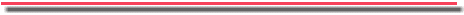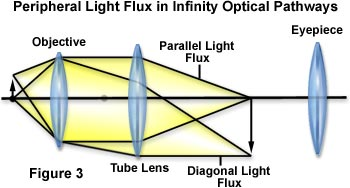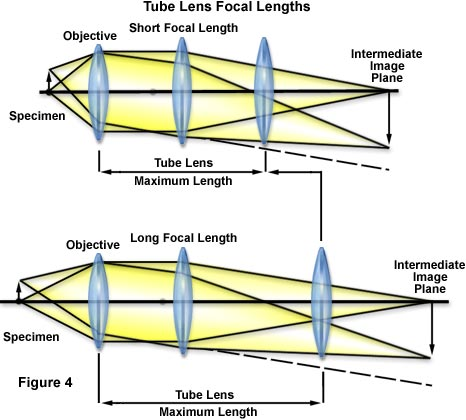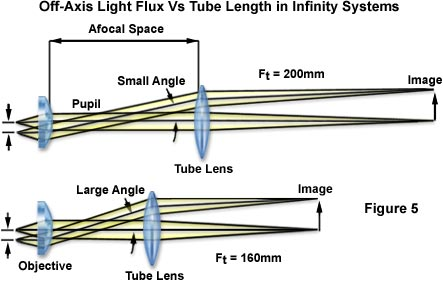CFI60 infinity opticsDesigned to correct chromatic aberrations over the entire field of view, CFI60 objectives produce images that are extremely sharp, of high contrast and have minimum of flare. Furthermore, their 60mm parafocal distance and larger objective diameter provide both longer working distances and higher numerical apertures. Longer working distances are particularly useful when inspecting thick samples.

Introduction

When typical microscopist speaks about Infinity Optics, they probably have this image
of a dream optical system that can do anything. Some say performance increases
when you use a microscope with an infinity optical system. So they conclude that if
it's not an infinity optical system, it is not performing at a high level.Are all manufacturers really trying hard to make this happen and to meet the
expectations of users? Is it true that infinity optics significantly improve system
flexibility, but is infinity optical performance always superior to finite optical systems?

Nikon CFI60 optical design team faced this proposition head on. They thoroughly
an optimum balance between optical performance and system flexibility. This
document will help you understand why an infinity optical system, for biological
applications, sets new performance standards incorporating a tube lens with a focal
length of 200 millimeters, an objective with a parfocal distance of 60 millimeters, and
an objective thread size of 25 millimeters.

Why is the Focal Length of the Tube Lens 200 millimeters?

In a finite optical system, after light from an object passes through the objective, it is
directed toward the primary image plane (often referred to as the intermediate image
plane, located at the eyepiece focal point) and converges there as illustrated in Figure
1.

In an infinity optical system, however, light becomes a flux of parallel rays after
passing through the objective and does not converge until after passing through the
tube lens as shown in Figure 2. This does not mean that an infinite distance can be
obtained after light passes the objective (up to the tube lens). After passing through
the objective, light from an object on the optical axis moves parallel to this axis along
the optical path. Light coming from the periphery of the object forms a flux of parallel
rays and advances at a diagonal angle to the optical axis as diagrammed in Figure 3,
presented below.Because of this, there are instances where these rays of light can no longer be
captured by the tube lens if the location of the tube lens is too far from the objective.
This causes the image around the edges of the field of view to become dark or blurred,
preventing the microscope from performing at its full potential. The term Infinity Optics
simply means that light becomes a flux of parallel rays after passing through the
objective, not that an infinite space is available inside the optical system.

If we are going to adopt infinity optics in order to further develop the microscope, we
will need to increase the distance between the objective and tube lenses as well as
increase the system flexibility. To lengthen this distance, we reduced the angle of the
flux of parallel rays outside the optical axis. It is generally thought that a longer focal
length for the tube lens will accomplish that, but this length has limitations.The magnification (M(o)) of the objective in an infinity optics microscope is obtained
using the formula:

Mo = tube lens focal length (Ft) / objective focal length (Fo)

where the tube lens focal length (F(t)) and objective focal length (F(o)) are described in
Figure 2. If the focal length of the tube lens is lengthened, the distance to the image
plane (at the eyepiece) will also increase with the longer focal length of the objective.
Naturally, this makes the size of the microscope larger. With this in mind, the
conclusion reached was that a focal length of 200 millimeters would be the most
appropriate for the tube lens. The focal lengths adopted by other manufacturers are
160 millimeters and 180 millimeters.

To obtain a same-size image from an object located far from the optical axis, the
longer focal length of the tube lens produces a smaller angle of light against the optical
axis. The light rays do not spread out so the distance between the tube lens and the
objective can be increased greatly enhancing the potential for system flexibility as
illustrated in Figure 4 below.This design has certain optical advantages. As shown in Figure 5, when tube lenses of
160 millimeters and 200 millimeters focal lengths are compared, the 200 millimeters
lens produces a flux of off-axis light rays with a smaller angle. In this context, light
rays passing through the phase ring in a phase contrast attachment, the DIC prism in
a Nomarski DIC attachment, or the dichroic mirror in an epi-fluorescence attachment,
produce smaller shifts between light elements parallel to the optical axis and those
diagonal to it, so that accessories work more efficiently. This is a big optical
advantage, and also a primary factor contributing to an improved level of contrast in
epi-fluorescence microscopy.Why is the Parfocal Distance of the Objective 60 millimeters?

Once the tube lens focal length was set to 200 millimeters, the parfocal distance of the
objective has to be increased from the standard 45 millimeters. As explained in the
section on tube length, the focal length of the objective is also increased in order to
preserve the same magnification, and since 45 millimeters does not provide optimum
space in this design, a high-quality image cannot be obtained. In practice, the CF N
Plan Apo 60x oil with a mechanical tube length of 160 millimeters, believed to be the
ultimate in finite objectives, is crowded with lenses in a limited space of 45 millimeters.
When this finite system is replaced with an infinite system and the objective is divided
into an objective and a tube lens, the focal length of the tube lens becomes the
equivalent of approximately 150 millimeters. On this basis, we can calculate the
parfocal distance to provide an optical performance which surpasses that of the finite
system as follows: The finite system objective parfocal distance is 45 millimeters; for a
tube lens focal length of 150 millimeters, the infinite system objective parfocal distance
is x; and the tube lens focal length is 200 millimeters. In solving this proportion, if 45 :
150 = x : 200, then x = 60 millimeters. Therefore, if the tube lens focal length is 200
millimeters, the optimum objective parfocal distance has to be 60 millimeters.

Using the calculations above the optimum parfocal distance for a tube length of 160
millimeters is 48 millimeters and for a tube length of 180 millimeters is 54 millimeters.
For microscope manufacturers who set the objective parfocal distance in their infinity
optics systems to 45 millimeters, then they are unable to exploit the full potential of
their objectives.

Since the working distance (WD) also increases to match the longer objective focal
length, manufacturers who use a parfocal distance of 45 millimeters are at a
disadvantage in their inability to utilize the longer working distance achieved by Nikon.
Using the Plan Apo 60x oil (N.A. 1.4) objective as a comparison, we see W.D.s by
manufacturer to be at least 50 percent less than those of Nikon. This shows that there
are differences in ability to accommodate various types of specimens as well as ease
of operation.

Common Infinity Correction Tube Lengths

 Manufacturer Tube Length (millimeters) Nikon 200 Olympus 180 Leica 200 Zeiss 164.5
Table 1

Low power lenses demand a specific size. If the magnification of the objective is 1x,
the "M(o) = F(t)/F(o)" formula used in the tube length section shows that the focal
length of the objective and that of the tube lens would have to be the same. In
Nikon's case, in order to perfect a tube lens focal length of 200 millimeters, a parfocal
distance of 45 millimeters would leave too little space in the design. By increasing this
distance to 60 millimeters, a magnification of 1x is obtainable and thanks to this
revolutionary change, an objective with a magnification as low as 0.5x has been
achieved. The lowest magnification offered by other manufacturers is 1.5x and none of
them has produced a 1x objective yet.

Why Use a 25 millimeters Objective Thread Size?

When the focal length of the tube lens is increased, the focal length of the objective
must also increase. There is a limit to the objective pupil diameter (effective diameter
remaining after the limits of the objective thread size), so a high numerical aperture
(N.A.) cannot be obtained. Thus, the N.A. of low-power lenses is critically affected. At
present, other manufacturers use a 20.32 millimeters thread size, but as mentioned
above, Nikon uses 25 millimeters and is able to attain high numerical apertures.
Originally, the brightness of photo lenses (F) was expressed with the formula:

F = f/D

where f is the lens focal length, and D is the effective diameter. Since the N.A. of a
microscope corresponds to the F value of a photo lens, the brightness can be
expressed with the formula:

F @ 1/(2N.A.)

The effective diameter needed to achieve a desired N.A. can thus be found using this
formula. In other words, the size of the pupil on an objective (effective diameter on the
exit side) is expressed as:

D = 2N.A. x f

For example, to find the effective diameter of the CFI Plan Apo 4x (N.A. 0.2), objective
with the highest (brightest) N.A.; given that the objective focal length is 50 millimeters,
and where the focal length of the tube lens is 200 millimeters, the following calculation

D = 2 x 0.2 x 50 = 20mm (optical diameter)

This shows that the conventional 20.32 thread size physically cannot be used. Pupil
diameters required for designing 4x objectives with a numerical aperture of 0.2 based
on 160 millimeters and 180 millimeters tube lengths are 16 millimeters and 18
millimeters respectively. This shows the kind of design problems faced by other
manufacturers when using a conventional 20.32 millimeters thread size. The actual
numerical apertures of the respective Plan Apo 4x objectives are 0.16. The N.A. for a
Nikon objective in this class is 0.20, which is the highest in the industry.

As shown, to obtain a high numerical aperture, a low-magnification objective requires a
large pupil diameter. The longer the focal length of the tube lens, the greater the
necessity to enlarge the thread size on the objective. Nikon has solved this problem by
choosing a 25 millimeters thread size for the CFI Infinity Optics system.

In Conclusion

We trust these explanations accompanied by specific examples have helped you to
understand why a tube lens of focal length 200 millimeters is considered optimum for
use in an infinity optical system and why higher optical specifications can be obtained
with an objective parfocal distance of 60 millimeters and a thread size of 25
millimeters. Through JIS and other conventional standards have been followed for
mechanical dimensions, the adoption of infinity optics itself has necessitated a
sacrifice in compatibility with conventional systems.

Thus, rather than be bound by conventional dimensions, Nikon felt that its true task
was to create products that users need for today's cutting edge microscopy
techniques. Innovations in engineering, manufacturing, quality control, inspection, and
production, have all contributed to the advent of Nikon's CFI60 series of optical
systems.